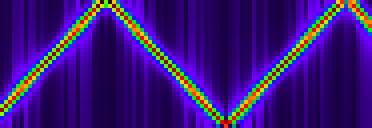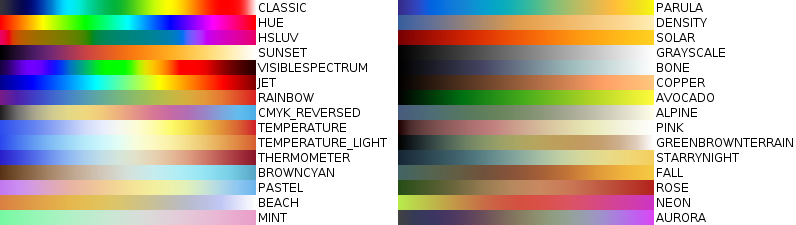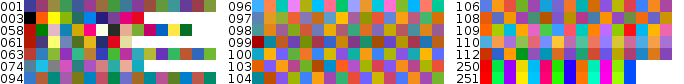{{ message }}
/ tensor Public

linear algebra for tensors with symbolic and numeric scalars

# idsc-frazzoli/tensor

Switch branches/tags
Could not load branches
Nothing to show

## Files

Failed to load latest commit information.
Type
Name
Commit time

# ch.ethz.idsc.tensorLibrary for tensor computations in Java, version `0.8.1`

The tensor library was developed with the following objectives in mind

• support for exact precision using integer fractions
• support for calculation with physical units
• suitable for use in safety-critical real-time systems
• API and string expressions inspired by `Mathematica`

Diverse projects rely on the tensor library:

## Features

• multi-dimensional arrays: scalars, vectors, matrices, n-linear forms, Lie-algebra ad-tensor, ...
• unstructured, nested tensors, for instance `{{1+2*I[A], -3/4}, {{5.678}, 9[kg*s^-1], 2[m^3]}}`
• scalars are real-, or complex numbers, from finite fields, or quantities with physical units
• values are encoded as exact integer fractions, in double precision, and as `java.math.BigDecimal`
• probability distributions for random variate generation: Binomial-, Poisson-, Exponential-distribution, etc.
• matrix functions `LinearSolve`, `SingularValueDecomposition`, `QRDecomposition`, etc.
• parametric functions `LinearInterpolation`, `BSplineFunction`, etc.
• window functions: Gaussian, Hamming, Hann, Blackman, etc.
• spectral analysis: `Fourier`, `SpectrogramArray`, etc.
• import from and export to `Mathematica`, `CSV`, and image files

## Examples

### Exact Precision

Solving systems of linear equations

```Tensor matrix = Tensors.matrixInt(new int[][] { { 2, -3, 2 }, { 4, 9, -3 }, { -1, 3, 2 } });
System.out.println(Pretty.of(Inverse.of(matrix)));

[
[   9/37    4/37   -3/37 ]
[ -5/111    2/37  14/111 ]
[   7/37   -1/37   10/37 ]
]```

Linear programming

```Tensor x = LinearProgramming.maxLessEquals( //
Tensors.vector(1, 1), // rewards
Tensors.fromString("{{4, -1}, {2, 1}, {-5, 2}}"), // matrix
Tensors.vector(8, 7, 2)); // rhs
System.out.println(x);

{4/3, 13/3}```

Null-space

```Tensor matrix = Tensors.fromString("{{-1/3, 0, I}}");
System.out.println(Pretty.of(NullSpace.of(matrix)));

[
[    1     0  -I/3 ]
[    0     1     0 ]
]```

Statistics

```Distribution distribution = HypergeometricDistribution.of(10, 50, 100);
System.out.println(RandomVariate.of(distribution, 20));
PDF pdf = PDF.of(distribution);
System.out.println("P(X=3)=" + pdf.at(RealScalar.of(3)));

{6, 5, 1, 4, 3, 4, 7, 5, 7, 4, 6, 3, 5, 4, 5, 4, 6, 2, 6, 7}
P(X=3)=84000/742729```

### Physical Quantities

The tensor library implements `Quantity`, i.e. numbers with physical units. Several algorithms are verified to work with scalars of type `Quantity`.

```Tensor matrix = Tensors.fromString( //
CholeskyDecomposition cd = CholeskyDecomposition.of(matrix);
System.out.println(cd.diagonal());
System.out.println(Pretty.of(cd.getL()));

[
[             1              0              0 ]
[ 1/2[m^-1*rad]              1              0 ]
[  1/3[kg*m^-1]   1[kg*rad^-1]              1 ]
]

The units of a quantity are chosen by the application layer. For instance, `Quantity.of(3, "Apples")` is valid syntax.

The tensor library contains the resource `/unit/si.properties` that encodes the SI unit system in the familiar strings such as `m`, `kg`, `s`, but the use of this convention is optional. The example below makes use of these provided definitions

```Scalar mass = Quantity.of(300, "g"); // in gram
Scalar a = Quantity.of(981, "cm*s^-2"); // in centi-meters per seconds square
Scalar force = mass.multiply(a);
System.out.println(force);
Scalar force_N = UnitConvert.SI().to(Unit.of("N")).apply(force);
System.out.println(force_N);

294300[cm*g*s^-2]
2943/1000[N]```

The arithmetic for the scalar type `Quantity` was developed in collaboration with the project `SwissTrolley+`.

### Miscellaneous

Tensors of rank 3

```Tensor ad = LieAlgebras.so3();
Tensor x = Tensors.vector(7, 2, -4);
Tensor y = Tensors.vector(-3, 5, 2);
System.out.println(ad.dot(x).dot(y)); // coincides with cross product of x and y

{{{0, 0, 0}, {0, 0, -1}, {0, 1, 0}}, {{0, 0, 1}, {0, 0, 0}, {-1, 0, 0}}, {{0, -1, 0}, {1, 0, 0}, {0, 0, 0}}}
{24, -2, 41}```

Functions for complex numbers

```System.out.println(Sqrt.of(RationalScalar.of(-9, 16)));

3/4*I```

Several functions support evaluation to higher than machine precision for type `DecimalScalar`.

```System.out.println(Exp.of(DecimalScalar.of(10)));
System.out.println(Sqrt.of(DecimalScalar.of(2)));

220255.6579480671651695790064528423`34
1.414213562373095048801688724209698`34```

The number after the prime indicates the precision of the decimal. The string representation is compatible with `Mathematica`.

Indices for the `set` and `get` functions start from zero like in C/Java:

```Tensor matrix = Array.zeros(3, 4);
matrix.set(Tensors.vector(9, 8, 4, 5), 2);
matrix.set(Tensors.vector(6, 7, 8), Tensor.ALL, 1);
System.out.println(Pretty.of(matrix));
System.out.println(matrix.get(Tensor.ALL, 3)); // extraction of the 4th column

[
[ 0  6  0  0 ]
[ 0  7  0  0 ]
[ 9  8  4  5 ]
]
{0, 0, 5}```

### Visualization

Image functions: `ArrayPlot`, `Spectrogram`

```Tensor data = Cos.of(Subdivide.of(0, 100, 2000).map(Series.of(Tensors.vector(0, 5, 1))));
Tensor image = Spectrogram.of(data, ColorDataGradients.VISIBLESPECTRUM);
Export.of(HomeDirectory.file("spectrogram.png"), ImageResize.nearest(image, 4));```

gives the image##### Predefined color gradients##### Predefined color lists## Integration

Specify `repository` and `dependency` of the tensor library in the `pom.xml` file of your maven project:

```<repositories>
<repository>
<id>tensor-mvn-repo</id>
<url>https://raw.github.com/idsc-frazzoli/tensor/mvn-repo/</url>
<snapshots>
<enabled>true</enabled>
<updatePolicy>always</updatePolicy>
</snapshots>
</repository>
</repositories>

<dependencies>
<dependency>
<groupId>ch.ethz.idsc</groupId>
<artifactId>tensor</artifactId>
<version>0.8.1</version>
</dependency>
</dependencies>```

The source code is attached to every release.linear algebra for tensors with symbolic and numeric scalars

v0.8.1 Latest
Nov 17, 2019

## Packages 0

No packages published

•
•
•
•